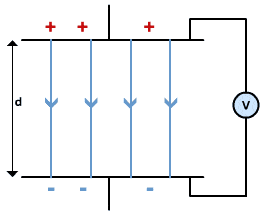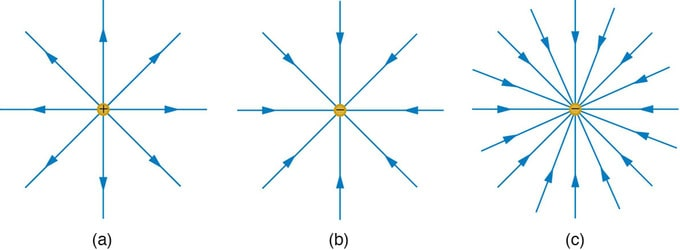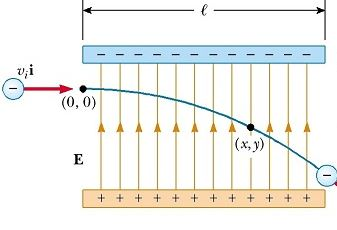Physic Tutorials

# Notes on electric field for Cambridge A level and UTME

Notes on electric field for Cambridge A level and UTME

Electric field is a region of space where an electric charge body/object experience a electric force.

Field of force

gravitational field – point mass

electric field – charge

magnetic field – current carrying conductor / charge

Field of force are always vector quantities because their field lines always show the direction. Electric field lines will always go in the direction from the positive to the negative region.Positive point charge : the field lines are radially outward and are always in the direction of the force

Negative point charge: the field lines are radially inwardPoint charge are spherical in shape and infinitesimal in nature

Electric field strenght: at a point in an electric field field is defined as the force per unit charge acting on a positive stationary charge at that point

E = f / q

cross multiply

F = qE

A ‘test charge’ should be positive. the direction it moves in then shows the direction of the electric field.

Uniform electric field

1.The field lines must be parallel

2.The field lines must be equally spaced

The electric field lines is from region of higher potential to a lower potential

The force acting on a charge inside a uniform electric field is constant at all point

The path of a charge inside a uniform electric field is always parabolic in path

Example of a uniform electric field is when you have two parallel plate ofE = v / d

E is the electric field strenght

v is the electric potential difference

d is the distance between the plates

Note that E can also be f / q

E = v / d = f / q

cross multiply

f.d = v . q

f . d = workdone

workdone = qv

Moving charge inside a uniform electric fieldBut a positive charge will deflect toward the negative charge in a parabolic path

Ek = qv

Ek = 1/2mv2

equate the two

1/2 m v2 = qvVel is the speed of the charge

v is the potential difference

m is the mass of the charge

q is the charge

Recommended: Solved questions on electric field

### Bolarinwa Olajire

A lecturer, Educationist, PhD student at FUNAAB, and a Blogger.